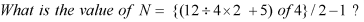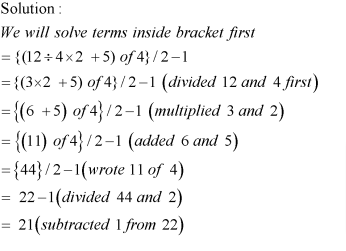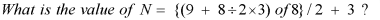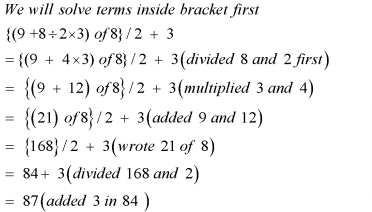How do we solve questions involving mathematical operations involving addition, subtraction, multiplication and division? The answer to this question is that we follow a particular order to solve the problem. This forms the basis to solve any kind of question.

The order is known as VBODMAS.

VBODMAS:  – It stands for (Vinculum- Bracket – Of –Division –Multiplication – Addition – Subtraction). This is an extended version of BODMAS.

V stands for vinculum or Bar (Bar which is used to show recurring numbers for e.g.) .

B stands for Bracket for e.g. () , {} ,[]

O stands for Of

D stands for Division  []

M stands for Multiplication [X]

S stands for subtraction [-]

Let us see some examples based on this.

Example 1:

What is the value of N=4+5-7?

Solution:

According to BODMAS, first addition should take place and then subtraction.

So, N =(4+5) -7 = 9-7 = 2

Example 2:

What is the value of N= {(4 +5) of4}/3 + 2?

Solution:

We will first solve the bracket, N = {(9) x 4}/3 + 2 = 12 + 2 = 14.

Let’s try out some more questions.

EXERCISE: –

Question 1:Question 2:

What is the value of N = {(18 ÷ 3 × 2 + 1) of 8}/2 + 5?

Solution:

We will solve the terms inside the bracket first

{(18 ÷ 3 × 2 + 1) of 8}/2 + 5

= {6 × 2 + 1) of 8}/2 + 5   ( divided 18 and 3)

= {(12 + 1) of 8}/2 + 5      (multiplied 6 and 2)

= {13 of 8}/ 2 + 5              ( added 12 and 1)

= (104)/2 + 5                      ( wrote 13 of 8)

= 52 + 5                              ( divide 104 and 2)

= 57                                    ( added 52 and 5)

Question 3:Question 4: Solve 2 × 6 + 3 – 4/2 – 5 + 20/5 × 3 + 50

Solution:  We have 2 × 6 + 3 – 4/2 – 5 + 20/5 × 3 + 50

= 12+3 – 4/2 – 5+20/5 × 3+50

= 12+3-2-5+20/5 × 3+50

= 12+3-2-5+4 ×3+50

= 12+3-2-5+12+50

= 15-2-5+12+50 = 77 – 7 = 70

Question 5: Solve  (3+3-5) × (15-5) × 10 – 99

Solution:

We have (3+3-5) × (15-5) × 10 – 99

=  (6 – 5) × (15 – 5) × 10 – 99

= 1 × (15 – 5) × 10 – 99 = 10 × 10 – 99 = 1

Get Posts Like This Sent to your Email
Updates for Free Live sessions and offers are sent on mail. Don't worry: we do not send too many emails..:)
Get Posts Like This Sent to your Email
Updates for Free Live sessions and offers are sent on mail. Don't worry: we do not send too many emails..:)